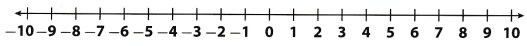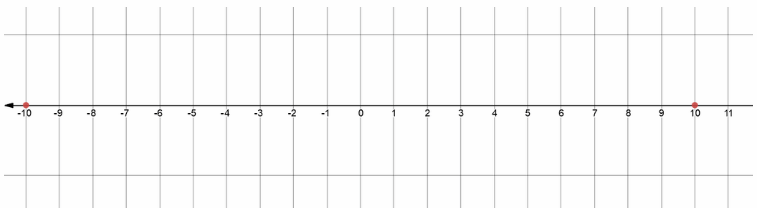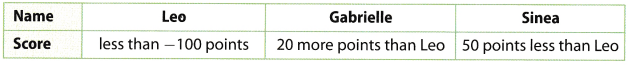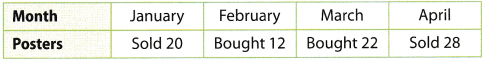# Texas Go Math Grade 6 Lesson 1.3 Answer Key Absolute Value

Refer to our Texas Go Math Grade 6 Answer Key Pdf to score good marks in the exams. Test yourself by practicing the problems from Texas Go Math Grade 6 Lesson 1.3 Answer Key Absolute Value.

## Texas Go Math Grade 6 Lesson 1.3 Answer Key Absolute Value

Reflect

Question 1.
Analyze Relationships Which pairs of numbers have the same absolute value? How are these numbers related?
Numbers which have same absolute value are opposites.
Therefore, their distance from 0 is equal!

Justify Reasoning Negative numbers are less than positive numbers. Does this mean that the absolute value of a negative number must be less than the absolute value of a positive number? Explain.
By definition of absolute value, it’s distance from 0 on a number Line.
Therefore, absolute value of a negative number mustn’t be
Less than the absolute value of a positive number!

Question 3.
Communicate Mathematical Ideas Explain why the absolute value of a number will never be negative.
Absolute value of number can not be negative since it is defined as a distance from 0.
Therefore, it can not be less than 0.

Question 4.
The temperature at night reached – 13°F Write an equivalent statement about the temperature using the absolute value of the number.
We can just write it like:
Temperature at night reached negative |-13|°F

Find each absolute value.

Question 5.
|-12| _________
The absolute value of – 12 equals 12
Therefore, we write:
|-12| = 12

Question 6.
|-91| _________
The absolute value of 91 equals 91
Therefore, we write:
|91| = 91

Question 7.
|-55| _________
The absolute value of – 55 equals 55
Therefore, we write:
|-55| = 55

Go Math Grade 6 Lesson 1.3 Absolute Value Answer Key Question 8.
|0| _________
The absolute value of 0 equals 0
Therefore, we write:
|0| = 0

Question 9.
|88| _________
The absolute value of 88 equals 88
Therefore, we write:
|88| = 88

Question 10.
|1| _________
The absolute value of 1 equals 1
Therefore, we write:
|1| = 1

Reflect

Question 11.
Analyze Relationships Use absolute value to describe the relationship between a negative credit card balance and the amount owed.
Let’s show it by an example:

We can simply say that if we owe $70 that means our credit card is$ – 70.
So, by usage of absolute value:

Amount we owe = absolute value of our balance( if we assume that balance is negative)

Question 1.
Vocabulary If a number is _____________, then the number is less than its absolute value. (Explore Activity 1)
If a number is negative, then we can say that number is smaller than its absolute value

Go Math Absolute Value Lesson 1.3 Practice Answer Key Question 2.
If Ryan pays his car insurance for the year in full, he will get a credit of $28. If he chooses to pay a monthly premium, he will pay a$10 late fee for any month that the payment is late. (Explore Activity 1 Example 1)

a. Which of these values could be represented with a negative number? Explain.
We can represent the $10 late fee as an absolute value. For example, we can say that$10 will be subtracted from his bank account for the mouth that the payment is late.

b. Use the number line to find the absolute value of the amount from part a. ____________Question 3.
Leo, Gabrielle, Sinea, and Tomas are playing a video game. Their scores are described in the table below. (Explore Activity 2)a. Leo wants to earn enough points to have a positive score. Does he need to earn more than 100 points or less than 100 points?
Leo has to earn more than 100 points.

b. Gabrielle wants to earn enough points to not have a negative score.
Does she need to earn more points than Leo or less points than Leo?
Gabrielle has 20 points more than Leo, So she has less than – 80 points.
This means that she has to be earn less points than Leo.

C. Sinea wants to earn enough points to have a higher score than Leo.
Does she need to earn more than 50 points or less than 50 points?
Sinea has 50 points less than Leo, so she has less than – 150 points.
This means that she has to earn more than 50 point to be greater than Leo.

Essential Question Check-In

Question 4.
When is the absolute value of a number equal to the number?
Absolute value of a number equal to the number only when the number is positive.

Question 5.
Financial Literacy Jacob earned $80 babysitting and deposited the money into his savings account. The next week he spent$85 on video games. Use integers to describe the weekly changes in Jacob’s savings account balance.
Jacob firstly gained $80 and then he spent$85 so this is equivalent to expression:
$80 –$85 = -$5 Now we can conclude that Jacob owes: |-$5| = $5 Jacob owes$5

Question 6.
Financial Literacy Sara’s savings account balance changed by $34 one week and by –$67 the next week. Which amount represents the greatest change?
We can describe greatest change by biggest distance from 0 on a number line
Therefore, we can use absolute value to calculate it:
|-$67| =$67
$|34| =$31
We know that number 67 is greater than 34 so now we can conclude that greatest change is – $67. Go Math 6th Grade Lesson 1.3 Answer Key Question 7. Analyze Relationships Bertrand collects movie posters. The number of movie posters in his collection changes each month as he buys and sells posters. The table shows how many posters he bought or sold in the given months.a. Which months have changes that can be represented by positive numbers? Which months have changes that can be represented by negative numbers? Explain. Answer: We can say that buying can be represented by positive value and selling can be represented by negative value. Therefore, we can write: March and February can be represented by positive number January and April can be represented by negative number b. According to the table, in which month did the size of Bertrand’s poster collection change the most? Use absolute value to explain your answer. Answer: Here, we take numbers from table and compare them and get: Number 12 is smaller than 20 which is smaller than 22 which is smaller than 28. So now we know that 28 is greatest which means that: Ir April the size of Bertrand’s poster changed the most! Question 8. Earth Science Death Valley has an elevation of – 282 feet relative to sea level. Explain how to use absolute value to describe the elevation of Death Valley as a positive integer. Answer: Here, we can use absoLute vaLue to determine depth of Death Valley: |- 282| = 282 feet We can say that Death Walley lies 282 feet below sea level. Algebra Lesson 1.3 6th Grade Math Absolute Value Question 9. Communicate Mathematical Ideas Lisa and Alice are playing a game. Each player either receives or has to pay play money based on the result of their spin. The table lists how much a player receives or pays for various spins.a. Express the amounts in the table as positive and negative numbers. Answer: We can express received value as positive number and paid value as negative number. b. Describe the change to Lisa’s amount of money when the spinner lands on red. Answer: When the spinner lands on red. Lisa has to pay$2.
We can say that her amount of money will change by -$2 Question 10. Financial Literacy Sam’s credit card balance is less than –$36. Does Sam owe more or less than $36? Answer: Since Sam has less tIin –$36 card balance
It means that we go left from – $36 on a number line! That implies that he owes more than$36.
Sam owes more than $36. Question 11. Financial Literacy Emily spent$55 from her savings on a new dress. Explain how to describe the change in Emily’s savings balance in two different ways.
We can say that her balance change is: – $55 Other way would be say that her balance decreased by$55.

H.O.T. Focus on Higher Order Thinking

Question 12.
Make a Conjecture Can two different numbers have the same absolute value? If yes, give an example. If no, explain why not.
Two different numbers can have same absolute value if they are opposites!
Yes, if they are opposites

Question 13.
Note that absolute value will always give positive value!
– |- 4| = – 4
Right side is of equation is similar:
|- (- 4)| = 4
It is not equal.